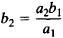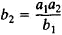# Three, Rule of

## Three, Rule of

a rule for solving arithmetic problems involving numbers in a proportion or an inverse proportion (seePROPORTIONALITY). Let us consider the two quantities x1 and x2, where x1 has the two values a1 and a2 and x2 has the two values b1 and b2. If the four values are in the proportion a1:b2 = a2:b2 or in the inverse proportion a1:b1 = b2:a2 and if a1, a2, and b1 are known, then the simple rule of three permits b2 to be found. In the case of a proportion we haveIn the case of an inverse proportion we haveWhat is called the compound rule of three permits the solution of proportion problems involving n quantities x1, x2, . . ., xn-1, xn (n > 2). Here, two values a1, a2, b1, b2, . . ., l1, l2 are known for each of the n-1 quantities x1, x2, . . ., xn-1, and only one value k1 is known for the quantity xn. The value k2 is to be found. In practice the compound rule of three consists in the successive application of the rule of three.

Site: Follow: Share:
Open / Close